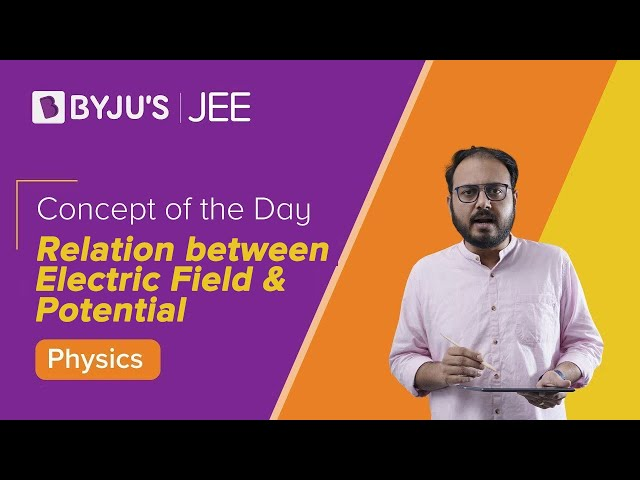# Relation between Electric Field and Electric Potential

The electric field exists if and only if there is an electric potential difference. If the charge is uniform at all points, however high the electric potential is, there will not be any electric field. Thus, the relation between electric field and electric potential can be generally expressed as – “Electric field is the negative space derivative of electric potential.”

## Electric Field and Electric Potential

The relation between the electric field and electric potential is mathematically given by

 $$\begin{array}{l}E=-\frac{dV}{dx}\end{array}$$

Where,

E is the Electric field.

V is the electric potential.

dx is the path length.

– sign indicates that the electric field is directed from higher potential to lower potential

### Video Explanation:### Direction of Electric Field

• If the field is directed from lower potential to higher then the direction is taken to be positive.
• If the field is directed from higher potential to lower potential then the direction is taken as negative.

## Electric Field and Electric Potential Relation

 Test charge Formula Electric gradient Positive $$\begin{array}{l}\frac{w}{q_{0}}=\int_{a}^{b}\vec{E}.d\vec{l}=V_{b}-V_{a}\end{array}$$ Higher as you go closer towards test charge. Negative $$\begin{array}{l}\frac{w}{q_{0}}=\int_{a}^{b}\vec{E}.d\vec{l}=V_{a}-V_{b}\end{array}$$ Higher as you go move away from test charge. Equipotential surface $$\begin{array}{l}\frac{w}{q_{0}}=\int_{a}^{b}\vec{E}.d\vec{l}=0\end{array}$$ Electric potential is perpendicular to Electric field lines.

## Electric Field and Electric Potential Relation Derivation

Let us study how to find the electric potential of the electric field is given. Work done in moving the test charge q0 from a to b is given by-

$$\begin{array}{l}W(\underset{a\rightarrow b}{q_{0}})= \int_{a}^{b}\vec{F}.d\vec{l} =q_{0}\int_{a}^{b}\vec{E}.d\vec{l}\end{array}$$

Where,

• F is the force applied
• dl is the short element of the path while moving it from a to b.

The force can be written as charge times electric field.

$$\begin{array}{l} =q_{0}\int_{a}^{b}\vec{E}.d\vec{l}\end{array}$$

Dividing both sides by test charge q0

$$\begin{array}{l}\frac{w}{q_{0}}=\int_{a}^{b}\vec{E}.d\vec{l}\end{array}$$

Work done by the test charge is the potential Va-Vb

$$\begin{array}{l}\int_{a}^{b}\vec{E}.d\vec{l} = V_{a}-V_{b}\end{array}$$

For equipotential surface, Va=Vb thus,

$$\begin{array}{l}\int_{a}^{b}\vec{E}.d\vec{l} = 0\end{array}$$

Hope you understood the relation and conversion between Electric Field and Electric potential.

Physics Related Topics:

Stay tuned with Byju’s for more such interesting articles. Also, register to “BYJU’S-The Learning App” for loads of interactive, engaging physics related videos and an unlimited academic assist.

Test Your Knowledge On Relation Between Electric Field And Electric Potential!